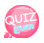# variable

Unlike other languages, php uses a \$(string) when declaring a variable.

ex) other program languages

int var;

var = 10;

in php

\$var = 10;

This is used.

When declaring variables, do not include special characters or numbers such as @, #,%, &,-.

When you want to specify two words as variables, you may use underscores or use camel notation.
For example, if you use an underscore
\$my_car
use this.
The camel notation is an English word for camel, meaning camel, and it is written like a camel's back.
The first letter of the first word is lowercase and only the first letter of the next word is capitalized.
For example, use
\$myCar as
above. The Camel notation is used a lot, so it is good to use the Camel notation.

```<?php
echo "How to declare a variable<br />";
\$num = 33;
\$num2 = 33.33;
\$studentName = "disney teyon kin";
echo "\\$num = \$num <br />";
echo "\\$num2 = \$num2 <br />";
echo "\\$studentName = \$studentName";
?>```

You may be wondering why you use \ before the variable name in the source above.
echo ""; If you write a variable name inside, the value of the variable is printed. However, if you prefix with \, the variable name is printed.
In other words, if you write \$num, you get 33, but if you write \ \$num, you get \$num.
If you have studied other programming languages, you may find it strange to assign variables.
Why would you think a float doesn't assign an integer to an integer?
php doesn't specify variable data type. The data type is automatically determined by the variable.
It's a bit comfortable. If you haven't experienced programming before, in other programming languages, when you put a phrase into a variable, the phrase before the declaration of the variable will be marked as numeric if it's a number, but if it's a mistake, php will do it automatically.
If different values ​​of the same variable name are entered, they will be replaced later.
For example, if you have the following source:

```<?php
\$num = 55;
echo "\$num";
\$num = 66.66;
echo "\$num";
?>```

\$num changes type from integer to real.
If you don't know what you're talking about, it makes sense later.
You don't have to waste your time why not.
Use gettype to get the data type of a variable.
For example echo gettype(\$num);
Enters the data type of \$num.

Integer is integer or int

floating-point number is double or float

Next is the auto cast

Mathematics = 100 points
English = 95 points
Science = program that calculates total score and average of 85 points
The average score is a cast of variables depending on the result of the operation.

```<?php
echo "Try to average 3 courses";
echo "<br />---------------------------- <br />";

\$math = 100;
\$english = 95;
\$science = 85;

\$sum = \$math + \$english + \$science; //Create a variable called \$sum and the value is the sum of 3 courses.
\$avg = \$sum / 3; //divided by 3 gives the average value of \$avg

echo " math point : \$math <br /> english point : \$english <br /> science point : \$science <br />";

echo "======================== <br />";

echo " total : \$sum <br />";

echo " average : \$avg <br />";

echo "======================== <br />";

echo " what is the type of \\$avg?  <br />";

echo gettype(\$avg);
?>```

Result

As you can see, \$avg has a double data typeInteger Overflow Casting ...

Exceeding the representation of integer values ​​(-2147483648 to 2147483647) results in a non-integer real number.
The example below uses a function called var_dump().
This function prints the data type and value.
Remember to use it a lot in practice. ^^;
So let's test it !!!

```<?php
echo "Overflow cast <br />";
echo "------------------------ <br />";
\$num = 2147483647; //put the largest number in the range
echo "variable \\$num output <br />";
var_dump(\$num);  // print values ​​and data types
echo "<br>.........[output as integer]<br /><br />";
\$num += 1;  // this means +1 on \$num, explained later.
echo "output of variable \\$num + 1  <br />";
var_dump(\$num);
echo "<br>.................[output float]";
?>```

Looking at the source above, when +1 was added to the existing num value, the data type was changed to float because it exceeded the limit of integer value.
Below is the resultI checked the results above and it still appears as an integer.
This is not an error. In the 32-bit environment, the integer representation is(-2147483648 to 2147483647).
In 64-bit environments, the integer representation is(-9223372036854775808 to 9223372036854775807).
Let's test again by changing 2147483647 to 9223372036854775807 in the code above.

```<?php
echo "Overflow cast <br />";
echo "------------------------ <br />";
\$num = 9223372036854775807; // put the largest number in the range.
echo "variable \\$num  output<br />";
var_dump(\$num);  // print values ​​and data types
echo "<br>.........[output as integer]<br /><br />";
\$num += 1;  // this means +1 on \$num, explained later.
echo "variable \\$num +1  output <br />";
var_dump(\$num);
echo "<br>.................[output as float]";
?>```

Below is the resultIf you are worried about seeing when you're taking a beginner's class, don't worry.
This tutorial goes through the mysql php interlock and login functions.
This is a course that I've already source coded once, glanced through the book, and once again I start to read the book twice, so that I can understand it properly.
Explain to others like this
I'm alone now and not just going over.
Interpret Variables in Strings
Be careful when entering variables in echo statements.
For example

```<?php
\$name = "asimo";
echo " \$nameis a robot   <br />";
echo " \$name is a robot <br />";
echo " {\$name}is a robot ... ";
?>```

Below is Result

In the first statement, the variable is recognized as \$name is, so no variable named \$name is declared, so nothing is displayed.

The second line is normal, normal output due to spacing.

The third is not spaced, but the output is printd with a definite distinction with {}